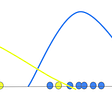# Supervised Random Projections made Lighter

Data scientists and Machine Learning practitioners are confronted with larger and larger datasets. Moore’s law as we know it is on its deathbed; we cannot rely on a sufficient increase in classical computing and storing capacity any longer. Novel hardware and smart algorithms are part of the answer. At LightOn, we devise innovative hardware and today’s blog entry will be about how we combine it with a new particularly efficient algorithm: Supervised Random Projections (SRP), by Karimi et al. (2018) .

## What if dimensionality reduction stopped ignoring labels?

Dimensionality reduction is becoming of primary importance as high-dimensional datasets become more common. In addition to easing memory and computing constraints, it enables visualization and often improves modelization. A well known technique, Principal Components Analysis (PCA), extracts the directions of highest variance in the data. They are expected to be, a priori, the most informative to make sense of the data. Mathematically, these directions are the eigenvectors of the covariance matrix of the input features. They are usually computed using a Singular Value Decomposition (SVD) of the feature matrix. However, since SVD does not scale well with matrix size, a surprisingly good method is to use a Random Projection instead, i.e. project the data to a lower dimension through the multiplication of the feature matrix with a rectangular random matrix. In many cases, results are almost as good as PCA, while the technique is computationally much lighter.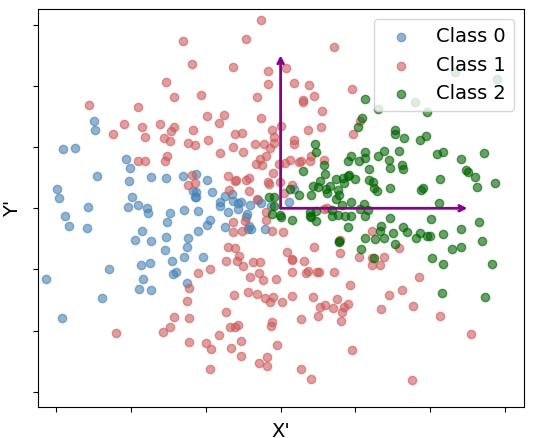Synthetic data generated on a disc in 3D space (left) can be reduced to 2D using PCA (right). The principal components (eigenvectors) are the purple vectors.

In the context of supervised learning, however, PCA is not ideal. Imagine you collect loads of data for various applications, but the task at hand only requires predicting a few variables. Not all your features will be informative for those particular variables, even though they are useful for other tasks. For instance, the last piece of clothing I bought helps a lot for recommending me clothes or even sportswear, but it is probably irrelevant to predicting my favorite food. Thus, the variance of a feature is not representative of its informativeness for the specific supervised task you are addressing. In more mathematical terms, you are now interested in the mutual information between your features and your target variables, not in the entropy of your features, i.e the information content irrespective of the task.

How do we quantify mutual information? The textbook definition requires knowing the true distribution of the data. This is quite unrealistic in the real world, though. To assess empirically the dependence between input features and target variables, we need to consider both linear and non linear relationships. Cross-correlation is a well-known metric, but it only accounts for linear dependencies. To also account for nonlinear ones, we must use slightly hairier math.

## Kernels to the rescue

A Reproducing Kernel Hilbert Spaces (RKHS) is a space of functions whose inner product can be computed using a kernel. A kernel is a function of two variables that computes a similarity score between them (a scalar). Given a kernel, there is always a corresponding RKHS, and vice-versa. If the kernel is positive definite, then it is also universal, which means that the corresponding RKHS contains all continuous functions. The RKHS is then also called universal.

To quantify both linear and non-linear relationships between features and targets, the authors use the Hilbert-Schmidt Independence Criterion (HSIC) . It first — theoretically — maps the data to two universal RKHSs, F for the features and G for the targets. It then measures the norm of the cross-covariance operator between F and G. Intuitively, that norm can be seen as the sum of the linear cross-correlation between all pairs of linear and non linear mappings in F and G of the features and targets . Because F and G are universal, this includes all continuous mappings. Thus, the HSIC cannot “miss” a correlation between the feature and target variables. The HSIC can’t be computed exactly, but there exists an empirical approximation for a dataset S of size n:

Here, K and L are the respective kernel (or Gram) matrices for the input features and the targets contained in S, and H is the centering matrix. The HSIC is 0 if the features and targets are independent, and takes a positive value that we can use as a measure of dependence — of any kind — otherwise.

## Supervised random projections: the technique

For dimensionality reduction, the goal is to map the data to a lower dimensional space, while keeping the most informative directions for predicting the targets. We denote X the feature matrix of size d x n, with the data points arranged in columns. The goal is then to find a projection matrix U of size d x k such that UᵀX maximizes the HSIC. Supervised PCA (SPCA)  shows that U is obtained as the first k eigenvectors of Q = XHLHXᵀ. SPCA is still a linear mapping of the data, though. You might want something more powerful to separate intricate classes. For that, you can use Kernel SPCA (KSPCA). It amounts to computing the first k eigenvectors of Q = HLHK, where K is the Gram matrix of the features computed with a kernel of your choice. SPCA and KSPCA are great, but both still use SVD, although on matrices of different sizes (d x d and n x n respectively). Once again, the computational cost of these methods can be prohibitive for large datasets, motivating the new SRP technique.

The authors of  show that, by decomposing L into ΨᵀΨ , then U = XHΨᵀ is the same as the SPCA solution up to a scaling and rotation. The projected data matrix is then Z = ΨHXᵀX. SRP can be extended to Kernel SRP (KSRP) by taking Z = ΨHK. Moreover, K can be approximated as K = ΦᵀΦ with random features to speed up computations further.

## Random projections with light

At LightOn, our first instance of light- based co-processors performs random projections. Our current Optical Processing Unit (OPU) uses the scattering of a laser beam passing through a thick diffusive medium to multiply a vector by a random matrix . Because we let the propagation of light through the diffusive medium perform the computation for us, its time and energy cost do not depend on the size of the input vector nor the size of the projection (up to about a million each). You can check this series to learn more about our vision.

For this blog entry, we wondered whether we could perform SRP and KSRP with an OPU. There are indeed a few constraints when using an OPU. First, the input must currently be binary for it to be encoded into our current input device. Second, the OPU doesn’t actually perform a real-valued linear random projection because the camera that reads the result only captures the intensity of the electromagnetic field. In mathematical terms: OPU(x)= |Rx|², where R is the — potentially giant — random matrix with complex-valued entries, and |.|² is to be taken element-wise. This feature map corresponds to the following kernel :

The informed reader might notice that this kernel is not universal. Still, it could be that universality is required only for far-fetched cases, and that this kernel works in most if not all realistic situations. Finally, the camera quantization is another non-linearity (each pixel is a uint8) added in the operation.

## SRP with LightOn’s OPU

We reproduced the experiments of , first with scikit-learn’s RBFSampler and then with one of our OPUs. There are two sets of experiments: 2D visualization of higher-dimensional datasets, or classification.

Here is how we proceeded for these experiments. In a classification setup, the labels are straightforward to encode to binary: either they already are, or one-hot encoding does the job. For the features, we used a simple random projection to a higher-dimensional space followed by a step function. Because pixel values are between 0 and 255, final values can get pretty large if we don’t constrain them in some way. That’s usually not a problem for classification, but can hurt visualizations. We use two methods: divide the values by 255, so they lie in the range [0,1], or pass them through a cosine function. The latter is closer to the original Random Fourier Features of .

The visualization experiments include three datasets. XOR and Spirals are toy datasets with two non linearly-separable classes. Each dataset has an intrinsic dimension of 2, to which 8 noise dimensions are added. Sonar is a real but tiny dataset from the UCI repository where samples are 60-dimensional.

SRP and KSRP work as expected. KSRP-OPU is not super convincing on Spirals but the OPU seems to implement SRP very well.

In the classification experiments, the authors reuse the XOR dataset along with two other datasets: UCI Ionosphere and MNIST. Following the paper, KSPCA and KSRP are not computed on MNIST (KSPCA leads to a memory error and KSRP is outperformed by SPCA and SRP). The classifier is 1-NN, i.e. nearest neighbors with a single neighbor. Dimensionality reduction techniques are of particular interest with this classifier, as Euclidean distance loses reliability in high dimensions. Here are the results:

We note a few things. First, our results are similar to those of the original paper. Second, even with a slightly different mathematical operation, the OPU can successfully implement SRP and KSRP. Actually, SRP-OPU performs better than both SRP and SPCA on XOR, which is interesting on its own. Third, SRP-OPU performs better than KSRP-OPU in the two cases where the comparison can be made. This effect is particularly striking on the Ionosphere dataset, for which the roles are almost perfectly reversed as compared to SRP and KSRP without OPU. The non-linearity of the OPU changes the analysis of the algorithm. The precise mechanism that affects the performance of SRP and KSRP with the OPU is left to further inquiries. Finally, the performance on MNIST is as expected: the curves for SPCA and SRP are the same as in the paper, and SRP-OPU perform almost on par with SRP.

## Give it a shot!

These results confirm that our OPU can implement those techniques successfully, especially SRP. The OPU shines with very high dimensional data: a projection is O(n), where n is the number of samples in your dataset, so essentially independent of the input and output dimensions. If your dataset has few features, like in these experiments, you can get away with a CPU or GPU. But when you reach tens of thousands of features, the CPU becomes unbearably slow and the GPU reaches its limits, whereas the OPU doesn’t even notice. Interested in trying our technology ? Please register here to have the opportunity to do so on LightOn Cloud.

## The author

François Boniface, Machine Learning R&D Engineer at Lighton AI Research

--

--

--

## More from LightOn

We are a technology company developing Optical Computing for Machine Learning. Our tech harvests Computation from Nature, We are at lighton.ai

Love podcasts or audiobooks? Learn on the go with our new app.

## Analysis of Transformer, Attention Mechanism and BERT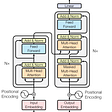## Introduction to PyCaret## Overcoming challenges when designing a fraud detection system## Machine Learning## How to not build a three-point ROC curve## Training Quantum Kernels for Machine Learning Using Qiskit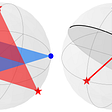## Deploy Rihanna lyric generation Model with Okteto Cloud## LightOn

We are a technology company developing Optical Computing for Machine Learning. Our tech harvests Computation from Nature, We are at lighton.ai

## Extrinsic & intrinsic rotation: Do I multiply from right or left?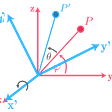## Hidden Markov Model — Evaluation Problem (Forward-backward Algorithm)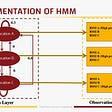## Working of Gaussian Mixture Model (GMM)